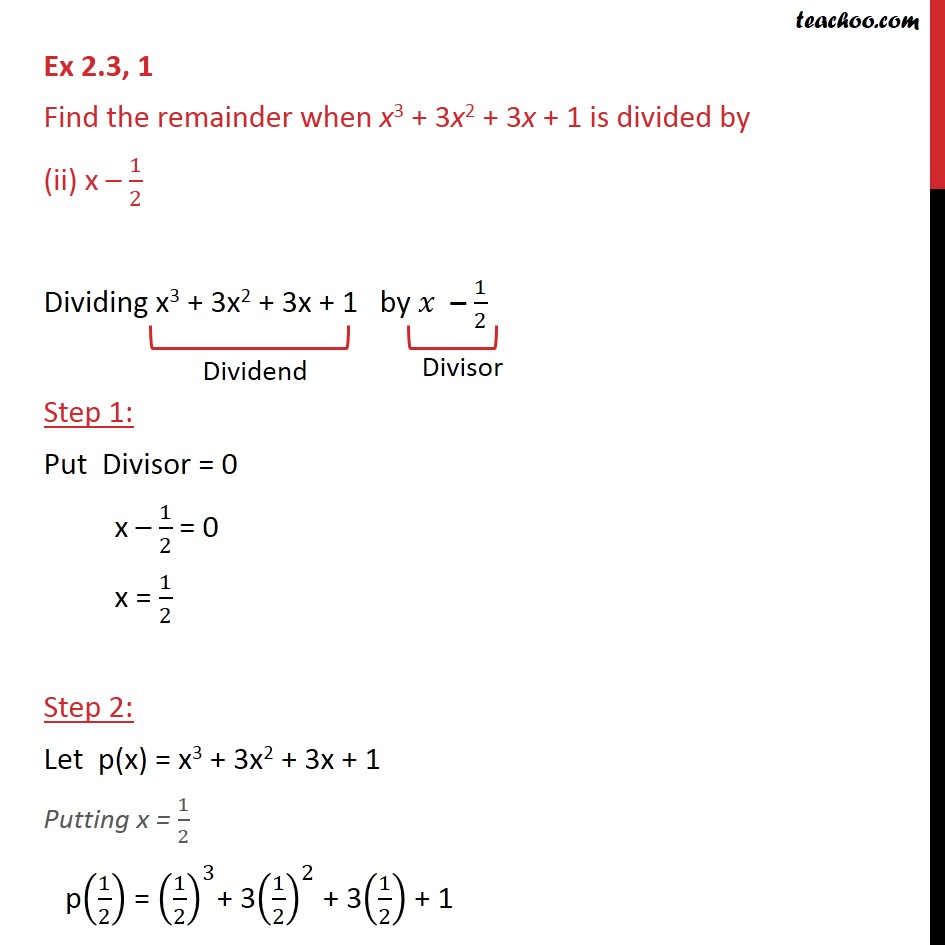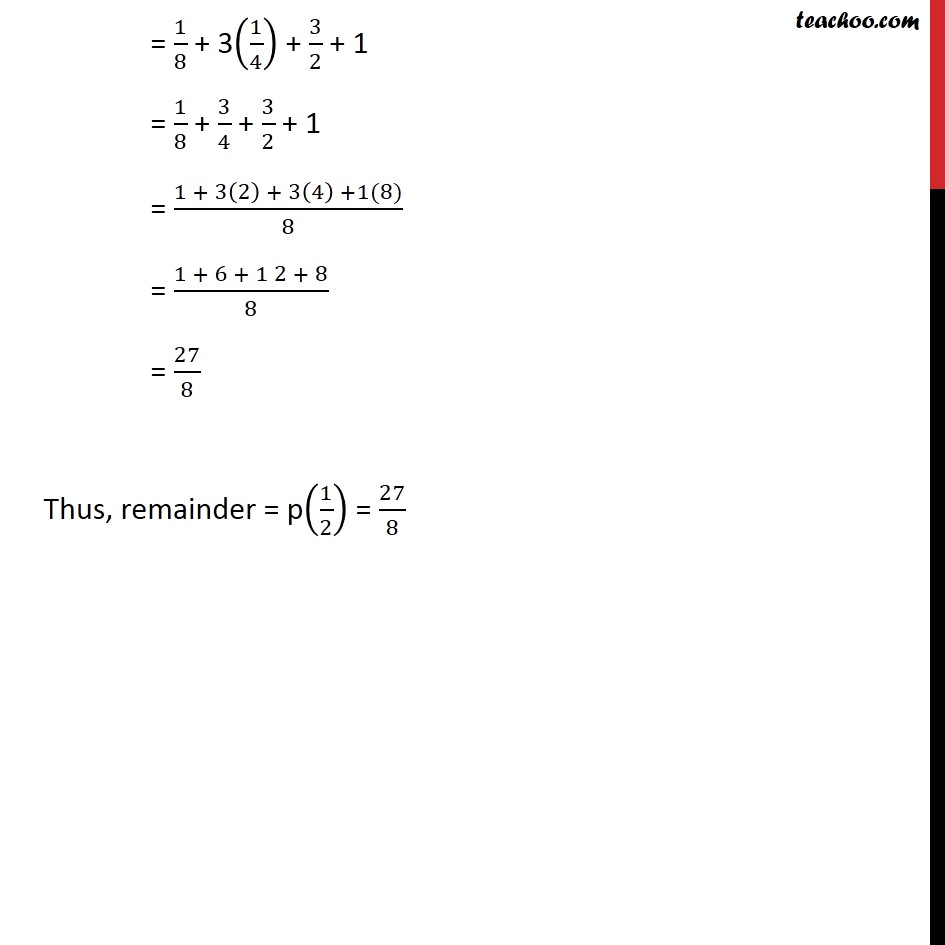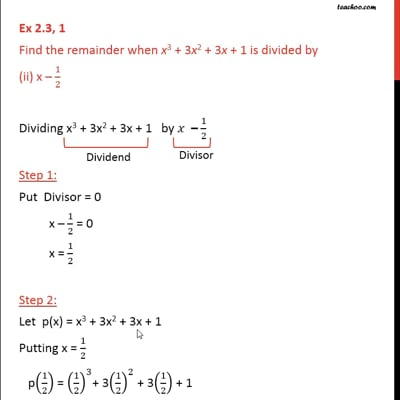Ex 2.3

Chapter 2 Class 9 Polynomials
Serial order wiseThis video is only available for Teachoo black users

Maths Crash Course - Live lectures + all videos + Real time Doubt solving!

### Transcript

Ex 2.3, 1 Find the remainder when x3 + 3x2 + 3x + 1 is divided by (ii) x – 1/2 Dividing x3 + 3x2 + 3x + 1 by 𝑥 – 1/2 Step 1: Put Divisor = 0 x – 1/2 = 0 x = 1/2 Step 2: Let p(x) = x3 + 3x2 + 3x + 1 Putting x = 1/2 p(1/2) = (1/2)^3+ 3(1/2)^2 + 3(1/2) + 1 = 1/8 + 3(1/4) + 3/2 + 1 = 1/8 + 3/4 + 3/2 + 1 = (1 + 3(2) + 3(4) +1(8))/8 = (1 + 6 + 1 2 + 8)/8 = 27/8 Thus, remainder = p(1/2) = 27/8